Dr. S. R. Lasker Library Online Catalogue

Normal view

# Introduction to mathematical statistics / Robert V. Hogg, Joseph W. McKean, Allen T. Craig.

Material type:TextLanguage: English Edition: 7th edDescription: 650 p. : ill. ; 24 cmISBN: 9780321795434 (hbk.); 9789332519114Subject(s): Mathematical statisticsDDC classification: 519.5 LOC classification: QA276 | .H59 2013Online resources: WorldCat details
Contents:
TOC Ch. 1. Probability and Distributions -- Ch. 2. Multivariable Distribution -- Ch. 3. Some Special Distributions -- Ch. 4. Some Elementary Statistical Inferences -- Ch. 5. Consistency and Limiting Distributions -- Ch. 6. Maximum Likelihood Methods -- Ch. 7. Sufficiency -- Ch. 8. Optimal Tests of Hypotheses -- Ch. 9. Inferences About Normal Models -- Ch. 10. Nonparametric and Robust Statistics -- Ch. 11. Bayesian Statistics.
Tags from this library: No tags from this library for this title.
Star ratingsAverage rating: 0.0 (0 votes)
Holdings
Item type Current library Collection Call number Copy number Status Date due Barcode Item holdsText
Reserve Section
Non-fiction 519.5 HOI 2014 (Browse shelf(Opens below)) C-1 Not For Loan 26643
Total holds: 0
##### Browsing EWU Library shelves, Shelving location: Reserve Section Close shelf browser (Hides shelf browser)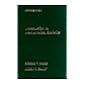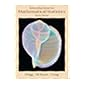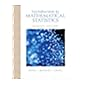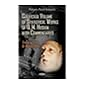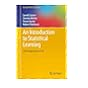519.5 HOI 1995 Introduction to mathematical statistics / 519.5 HOI 2005 Introduction to mathematical statistics / 519.5 HOI 2005 Introduction to mathematical statistics / 519.5 HOI 2014 Introduction to mathematical statistics / 519.5 HOT 1978 Introduction to mathematical statistics / 519.5 HUC 2013 Collected volume of statistical works of Q.M. Husain with commentaries / 519.5 INT 2017 An introduction to statistical learning :

Includes bibliographical references (669-672) and index.

TOC Ch. 1. Probability and Distributions --
Ch. 2. Multivariable Distribution --
Ch. 3. Some Special Distributions --
Ch. 4. Some Elementary Statistical Inferences --
Ch. 5. Consistency and Limiting Distributions --
Ch. 6. Maximum Likelihood Methods --
Ch. 7. Sufficiency --
Ch. 8. Optimal Tests of Hypotheses --
Ch. 9. Inferences About Normal Models --
Ch. 10. Nonparametric and Robust Statistics --
Ch. 11. Bayesian Statistics.

AS MPS

Tahur Ahmed

There are no comments on this title.